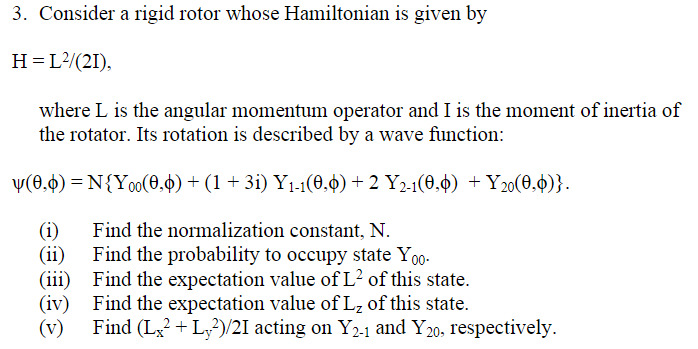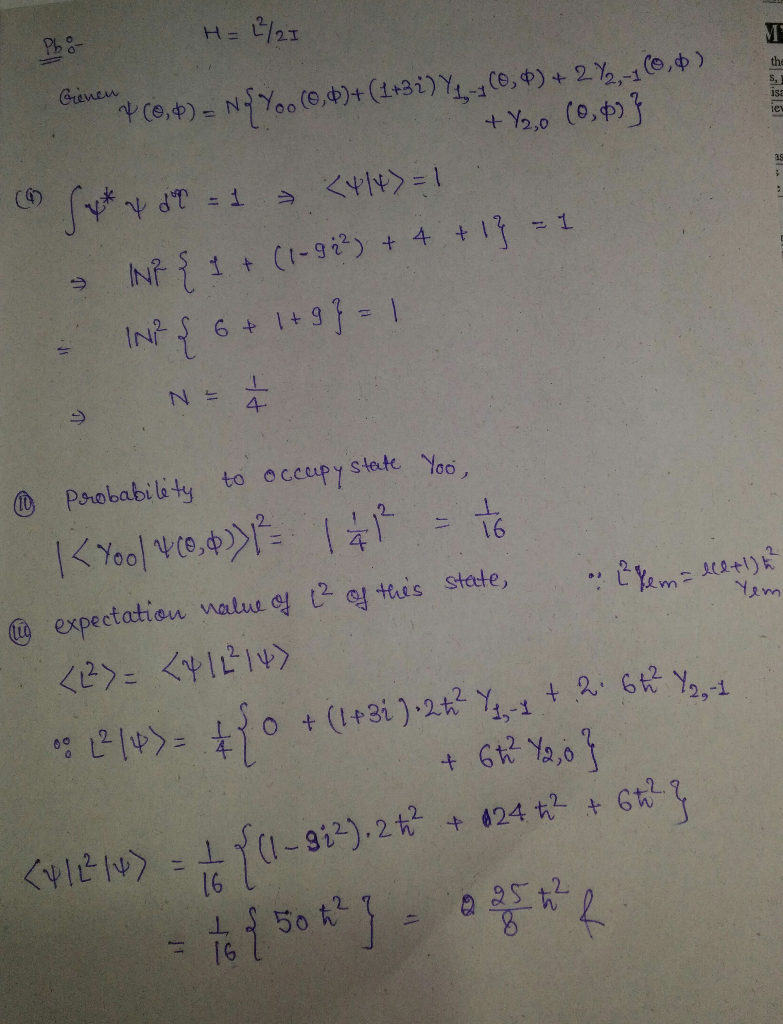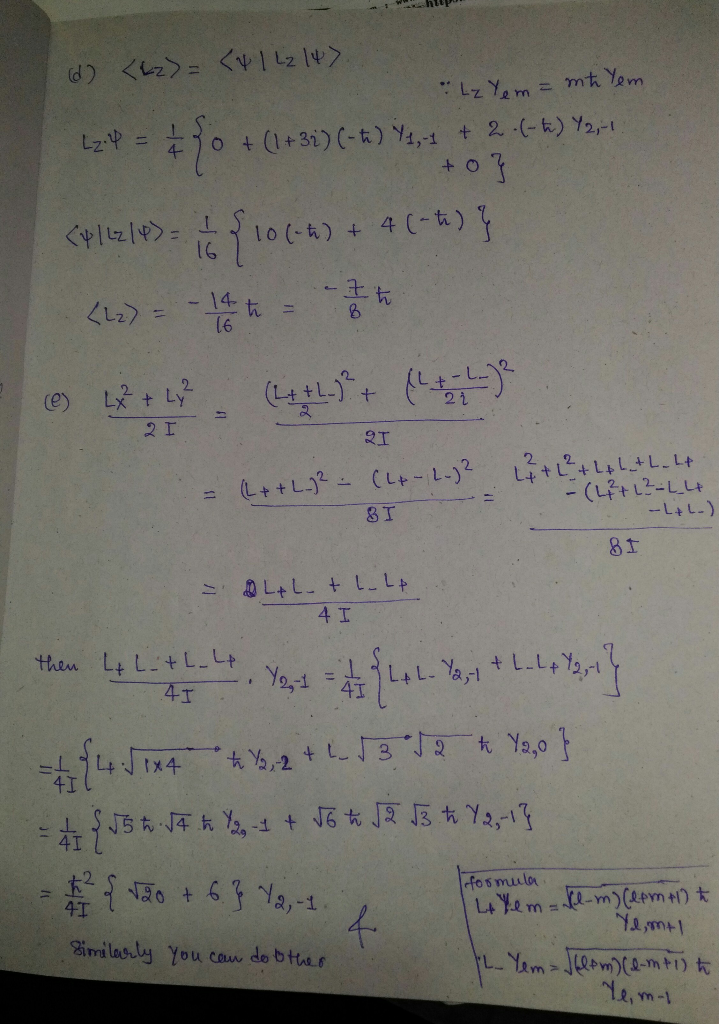# 3. Consider a rigid rotor whose Hamiltonian is given by H L2(21) where L is the angular momentum operator and I is the...3. Consider a rigid rotor whose Hamiltonian is given by H L2(21) where L is the angular momentum operator and I is the moment of inertia of the rotator. Its rotation is described by a wave function: (0, N{Yo0(0,6)(1 3i) Y1-1(0,6) 2 Y21(0.0) Y20(0.) Find the normalization constant, N. (i) Find the probability to occupy state Yo0- (ii Find the expectation value of L2 of this state (iii Find the expectation value of L2 of this state (iv) Find (L2L2/21 acting on Y2-1 and Y20, respectively (v)##### Add Answer of: 3. Consider a rigid rotor whose Hamiltonian is given by H L2(21) where L is the angular momentum operator and I is the...
More Homework Help Questions Additional questions in this topic.